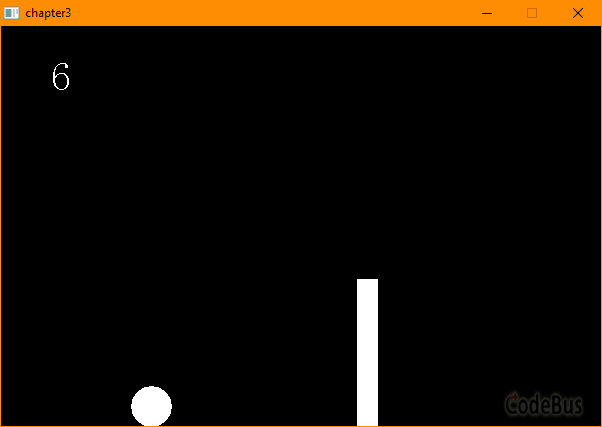# 童晶``````#include <graphics.h>
#include <conio.h>
#include <stdio.h>
int main()
{
float width,height,gravity; // 游戏画面大小、重力加速度
float rect_left_x,rect_top_y,rect_width,rect_height,rect_vx; // 方块障碍物的相关参数
int score = 0; // 得分
int isBallOnFloor = 1; // 小球是否在地面上，避免重复起跳

width = 600;  // 游戏画面宽度
height = 400; // 游戏画面高度
gravity = 0.6;  // 重力加速度
initgraph(width, height); // 新建一个画布

ball_x = width/4; // 小球x位置
ball_vy = 0;  // 小球初始y速度为0

rect_height = 100; // 方块高度
rect_width = 20; // 方块宽度
rect_left_x = width*3/4; // 方块左边x坐标
rect_top_y = height - rect_height; // 方块顶部y坐标
rect_vx = -3; // 方块x方向速度

while(1) // 一直循环
{
if (kbhit()) // 当按键时
{
char input = _getch(); // 获得输入字符
if (input==' ' && isBallOnFloor==1) // 当按下空格键，并且小球在地面上时
{
ball_vy = -17; // 给小球一个向上的速度
isBallOnFloor = 0; // 表示小球不在地面了，不能重复起跳
}
}

ball_vy = ball_vy + gravity;  // 根据重力加速度更新小球y方向速度
ball_y = ball_y + ball_vy;    // 根据小球y方向速度更新其y坐标
if (ball_y >= height-radius)  // 如果小球落到地面上
{
ball_vy = 0;  // y速度为0
isBallOnFloor = 1; // 表示小球在地面上
}

rect_left_x = rect_left_x + rect_vx; // 方块向左移
if (rect_left_x <= 0) // 如果方块跑到最左边
{
rect_left_x = width; // 在最右边重新出现
score = score + 1; // 得分+1
rect_height = rand() % int(height/4) + height/4; // 设置随机高度
rect_vx = rand()/float(RAND_MAX) *4 - 7; // 设置方块随机速度
}
// 如果小球碰到方块
if ((rect_left_x <= ball_x + radius)
&& (rect_left_x + rect_width >= ball_x - radius)
&& (height - rect_height <= ball_y + radius) )
{
Sleep(50); // 慢动作效果
score = 0; // 得分清零
}

cleardevice();  // 清空画面
// 画方块
fillrectangle(rect_left_x, height - rect_height, rect_left_x + rect_width,height);
TCHAR s; // 定义字符串数组
_stprintf(s, _T("%d"),  score); // 将score转换为字符串
settextstyle(40, 0, _T("宋体")); // 设置文字大小、字体
outtextxy(50, 30, s); // 输出得分文字
Sleep(10);  // 暂停10毫秒
}
closegraph();
return 0;
}``````

视频教程可以参考这里：https://zhuanlan.zhihu.com/p/186282897